# Work done by variable force

• MatinSAR
Yeah, you replace it with whatever variable you want.the equation of path is x=(2a) sinθ and y=(a) cosθWhat is ##\theta## here? It can't be the argument parameter of the polar representation of (x,y) since we would deduce ##\tan^2(\theta)=\frac 12##. So I assume it is an arbitrary parameter unrelated to polar coordinates. But then, where do the integration bounds come from?I see no diagram.f

#### MatinSAR

Homework Statement
How can I find work done by variable force using F.dr When the equation of path is x=(2a) sinθ and y=(a) cosθ and F=(x^2) y i + x(y^2) j? If you can guide me how to write the integral relation so that I can calculate it, I would be grateful.
Relevant Equations
W=Int(F.dr)
Can anyone tell me if my answer is wrong ?!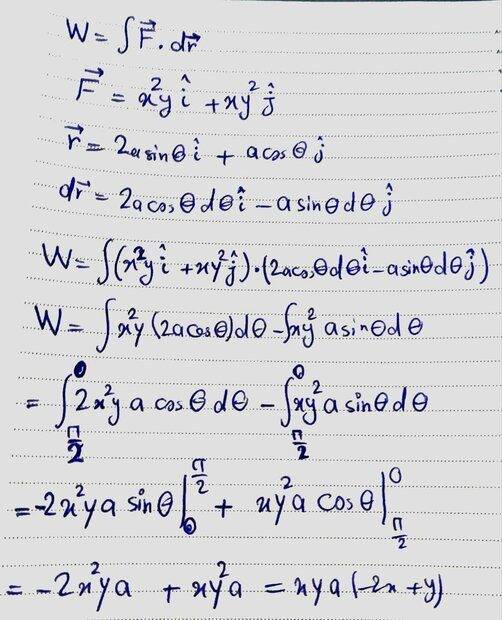Last edited:
What is F equal to in terms of "a" and ##\theta##?

•topsquark and MatinSAR
Just to clear things up:

$$r(t) = x ~\mathbf{\hat{i} } + y ~\mathbf{\hat{j} } = 2 a \sin t ~ \mathbf{ \hat{i}} + a \cos t ~ \mathbf{\hat{j}}$$

$$F(t) = x^2 y ~\mathbf{ \hat{i} } + x y^2 ~\mathbf{ \hat{j} }= ( 2 a \sin t )^2 a \cos t ~\mathbf{ \hat{i} } + 2 a \sin t (a \cos t )^2 ~\mathbf{ \hat{j} }$$

Correct?

Last edited:
•topsquark and MatinSAR
Assuming that you are asked to find the work done by the force from ##t=0## to some arbitrary time ##t##, you can write $$W=\int_0^t \mathbf{F}(t')\cdot d\mathbf{r}=\int_0^t \mathbf{F}(t')\cdot \frac{d[\mathbf{r}(t')]}{dt'}dt'$$which you can easily integrate because you are given both the force and the position vector as functions of time.

•topsquark, erobz and MatinSAR
What is F equal to in terms of "a" and ##\theta##?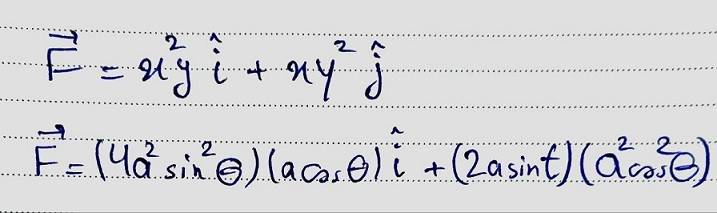Just to clear things up:

$$r(t) = x \, \rm{\hat i} + y \, \rm{\hat j} = 2 a \sin t \, \rm{ \hat i} + a \cos t \, \rm{\hat j}$$

$$F(t) = x^2 y \, \rm{\hat i }+ x y^2 \, \rm{\hat j} = ( 2 a \sin t )^2 a \cos t \, \rm{\hat i} + 2 a \sin t (a \cos t )^2 \, \rm{\hat j }$$

Correct?
Yes.

•topsquark
Assuming that you are asked to find the work done by the force from ##t=0## to some arbitrary time ##t##, you can write $$W=\int_0^t \mathbf{F}(t')\cdot d\mathbf{r}=\int_0^t \mathbf{F}(t')\cdot \frac{d[\mathbf{r}(t')]}{dt'}dt'$$which you can easily integrate because you are given both the force and the position vector as functions of time.
Thanks a lot. I have seen a formula like this before.
I think my mistake is that I did not consider the unit vector tangent to the direction of movement.
Can you please tell me what is that t' ?!

And we have position vector as functions of θ ...

Thanks a lot. I have seen a formula like this before.
I think my mistake is that I did not consider the unit vector tangent to the direction of movement.
Can you please tell me what is that t' ?!
Its just a "dummy variable" so the variable of integration is not the integration limits variable. Alternatively you could leave the force and position in terms of ##t## and switch the limits to ##0 ## and ##t'## or ##0## and ##t_f## is quite common

•topsquark and MatinSAR
That ##t'## is a dummy variable of integration that distinguishes it from "any time ##t##" that appears as an upper limit. It's a rigorous way to show what one is integrating with respect to. For example, $$\int_0^t 2t'dt'=\left . t'^2 \right |_0^t=t^2.$$

•topsquark, erobz and MatinSAR
Homework Statement:: How can I find work done by variable force using F.dr When the equation of path is x=(2a) sinθ and y=(a) cosθ and F=(x^2) y i + x(y^2) j? If you can guide me how to write the integral relation so that I can calculate it, I would be grateful.
Relevant Equations:: W=Int(F.dr)

Can anyone tell me if my answer is wrong ?!

View attachment 317187
Please don't edit your original post to change the statement of the problem. In the original version, the force and postion vectors were explicit functions of time. Now they are not. What is going on?

•topsquark and MatinSAR
Please don't edit your original post to change the statement of the problem. In the original version, the force and postion vectors were explicit functions of time. Now they are not. What is going on?
I am so sorry I didn't know that editing the original post is not allowed ...
I was trying to write theta but I couldn't. I have sent a picture and in that picture x and y are functions of θ.

I am so sorry I didn't know that editing the original post is not allowed ...
I was trying to write theta but I couldn't. I have sent a picture and in that picture x and y are functions of θ.
It would be better if you could just learn to use the math formatting available to you on the site: Latex

•topsquark and MatinSAR
Assuming that you are asked to find the work done by the force from ##t=0## to some arbitrary time ##t##, you can write $$W=\int_0^t \mathbf{F}(t')\cdot d\mathbf{r}=\int_0^t \mathbf{F}(t')\cdot \frac{d[\mathbf{r}(t')]}{dt'}dt'$$which you can easily integrate because you are given both the force and the position vector as functions of time.
Can I replace θ with t' ?!

Can I replace θ with t' ?!
Yeah, you replace it with whatever variable you want.

•topsquark and MatinSAR
the equation of path is x=(2a) sinθ and y=(a) cosθ
What is ##\theta## here? It can't be the argument parameter of the polar representation of (x,y) since we would deduce ##\tan^2(\theta)=\frac 12##. So I assume it is an arbitrary parameter unrelated to polar coordinates. But then, where do the integration bounds come from?
I see no diagram.
Can I replace θ with t' ?!
I guess you mean replace t' with ##\theta##.

•MatinSAR and topsquark
given both the force and the position vector as functions of time.
Don't know what post #1 said originally (helps if the first responder always uses 'reply'), but it now gives force as a function of position.
@MatinSAR , to use kuruman's approach you will need to find force as a function of theta.

•MatinSAR and topsquark
$$\vec{F}=2a^3\sin{\theta}\cos{\theta}(2\sin{\theta}\ \hat{i}+\cos{\theta}\ \hat{j})$$
$$\vec{dr}=a(2\cos{\theta}\ \hat{i}-\sin{\theta}\ \hat{j})d\theta$$

•MatinSAR
What is θ here?
Thank you ...

x and y are the equations of a part of an ellipse. We are going from a to c.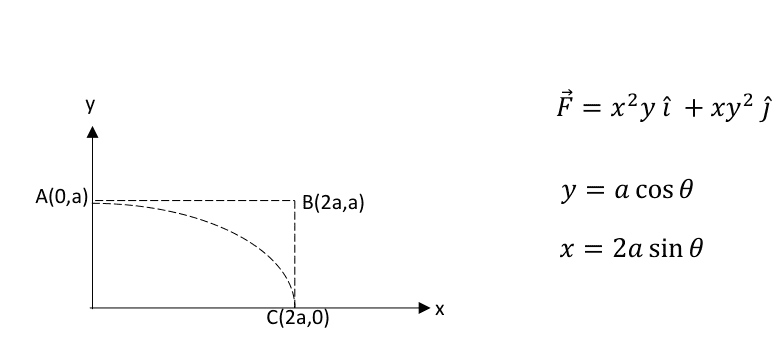I have tried to use that integral but I failed and My teacher said that my answer is wrong again ...
He suggusted using polar coordinates to answer the question.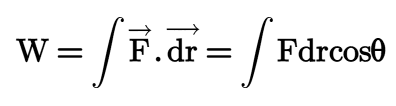We can convert F to polar coordinates like this : https://www.physicsforums.com/threads/work-done-by-variable-force.1047266/post-6821666
Then we can find it's magnitude ...
Magnitude of dr is rdθ ...

Last edited:
Draw a line from the origin to any point on the ellipse. Try to figure out what angle ( w.r.t the axes) ##\theta## must be such that the ##x## coordinate is given by ##2 a \sin \theta##.

•MatinSAR
Draw a line from the origin to any point on the ellipse. Try to figure out what angle ( w.r.t the axes) ##\theta## must be such that the ##x## coordinate is given by ##2 a \sin \theta##.
I have tried to find a similar example in many Classical Dynamics and Physics books but I didn't find anything similar ... I have searched the web also ... Do you know a bookthat has a question like this ?!

I have tried to find a similar example in many Classical Dynamics and Physics books but I didn't find anything similar ... I have searched the web also ... Do you know a bookthat has a question like this ?!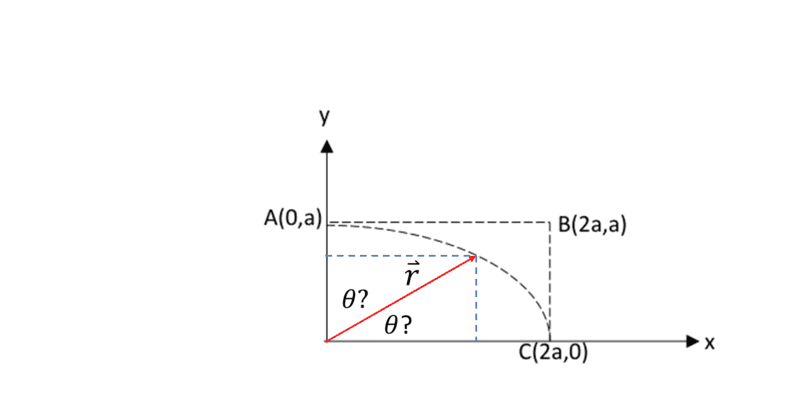## \vec r ## is the position vector. Which ##\theta## ( above ## \vec r ## or below it ) reconciles with ##x = 2 a \sin \theta## ?

Last edited:
•MatinSAR
Thank you but what do you mean by this ?! I didn't understand.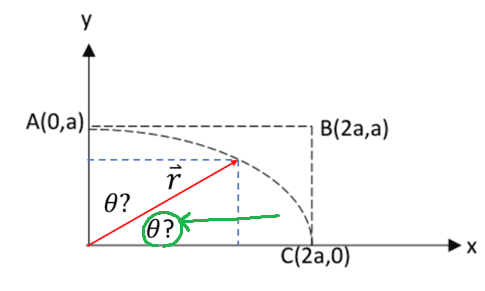This one should be Theta ...

Thank you but what do you mean by this ?! I didn't understand.

View attachment 317261
This one should be Theta ...
Using that angle if you plug in ##\theta = 0 ## what does ##x## equal?

•MatinSAR
Using that angle if you plug in ##\theta = 0 ## what does ##x## equal?
Theta=0 means x=0 and y=a

Theta=0 means x=0 and y=a
Does that seem correct to you?

•MatinSAR
Does that seem correct to you?
According to the diagram no it's wrong ... so the other one is theta and I have chose the wrong one ...

•erobz
I have tried to find a similar example in many Classical Dynamics and Physics books but I didn't find anything similar ... I have searched the web also ... Do you know a bookthat has a question like this ?!
I recommend that you try to reason your way through this one instead of trying to find one like it. You'll end up getting higher test scores.

•MatinSAR
Forget ##\theta## as a variable. Try this. You have $$x=2a\sin\theta~;~~2y=2a\cos\theta.$$It follows that $$x^2+4y^2=4a^2 \implies xdx+4ydy=0 \implies dx=-\frac{4y}{x}dy$$ Now $$\mathbf{F}\cdot d\mathbf{r}=(x^2y~\mathbf{\hat x}+xy^2~\mathbf{\hat y})\cdot(dx~\mathbf{\hat x}+dy~\mathbf{\hat y}) =x^2ydx+xy^2dy.$$ Substitute the expression for ##dx## and integrate over ##y##.

•MatinSAR
If you are going from A to C, the limits of integration are from ##\theta=0## to ##\theta = \frac{\pi}{2}##. From the equations for F and dr that I gave in post #16, what is the equation for F dotted with dr?

•MatinSAR
I recommend that you try to reason your way through this one instead of trying to find one like it. You'll end up getting higher test scores.
Thank you but I was looking for a similar question that has an answer to understand how to solve these kind of questions.

Forget ##\theta## as a variable. Try this. You have $$x=2a\sin\theta~;~~2y=2a\cos\theta.$$It follows that $$x^2+4y^2=4a^2 \implies xdx+4ydy=0 \implies dx=-\frac{4y}{x}dy$$ Now $$\mathbf{F}\cdot d\mathbf{r}=(x^2y~\mathbf{\hat x}+xy^2~\mathbf{\hat y})\cdot(dx~\mathbf{\hat x}+dy~\mathbf{\hat y}) =x^2ydx+xy^2dy.$$ Substitute the expression for ##dx## and integrate over ##y##.
Thanks a lot !
I have done this before but I didn't know anything about the substitution. I will try thank you ...
If you are going from A to C, the limits of integration are from ##\theta=0## to ##\theta = \frac{\pi}{2}##. From the equations for F and dr that I gave in post #16, what is the equation for F dotted with dr?
Thank you.
I will try to answer the problem using this method and compare with the method that Mr.kuruman said ...

•kuruman
That's worrying. If you do try that be careful not to use θ for both purposes. Either take the polar coordinates to be (r,φ) or change the given parameter from θ to t, say. I say it is worrying because it makes me wonder whether your teacher has blundered.
Thank you but what do you mean by this ?! I didn't understand.

View attachment 317261
This one should be Theta ...
No, neither of them matches the given theta. The one you circled would give ##x=r\cos(\theta), y=r\sin(\theta), y/x=\tan(\theta)##. But you are given ##y/x= \frac 12\cot(\theta)##.
If you draw a line from the origin to a point half way along the horizontal dashed blue line, the angle between that and the y-axis is the given theta.

•MatinSAR
If you are going from A to C, the limits of integration are from ##\theta=0## to ##\theta = \frac{\pi}{2}##. From the equations for F and dr that I gave in post #16, what is the equation for F dotted with dr?
Is it true ?!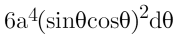Forget ##\theta## as a variable. Try this. You have $$x=2a\sin\theta~;~~2y=2a\cos\theta.$$It follows that $$x^2+4y^2=4a^2 \implies xdx+4ydy=0 \implies dx=-\frac{4y}{x}dy$$ Now $$\mathbf{F}\cdot d\mathbf{r}=(x^2y~\mathbf{\hat x}+xy^2~\mathbf{\hat y})\cdot(dx~\mathbf{\hat x}+dy~\mathbf{\hat y}) =x^2ydx+xy^2dy.$$ Substitute the expression for ##dx## and integrate over ##y##.
Can I send a picture of my work ?! The final answer is unusual ...

That's worrying. If you do try that be careful not to use θ for both purposes. Either take the polar coordinates to be (r,φ) or change the given parameter from θ to t, say. I say it is worrying because it makes me wonder whether your teacher has blundered.

No, neither of them matches the given theta. The one you circled would give ##x=r\cos(\theta), y=r\sin(\theta), y/x=\tan(\theta)##. But you are given ##y/x= \frac 12\cot(\theta)##.
If you draw a line from the origin to a point half way along the horizontal dashed blue line, the angle between that and the y-axis is the given theta.
Thanks ...
Is it true ?!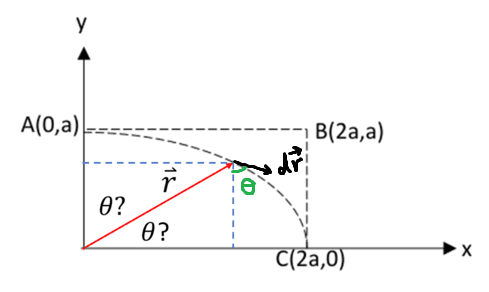Can I send a picture of my work ?! The final answer is unusual ...
LaTeX is preferable, but if your work is too involved, you can post a picture as long as it is clearly legible and right side up. Use the "Attach files" link, lower left.

•MatinSAR
Is it true ?!
View attachment 317282
I don't get the factor as 6. Did you handle the signs correctly? The rest looks fine.

•MatinSAR